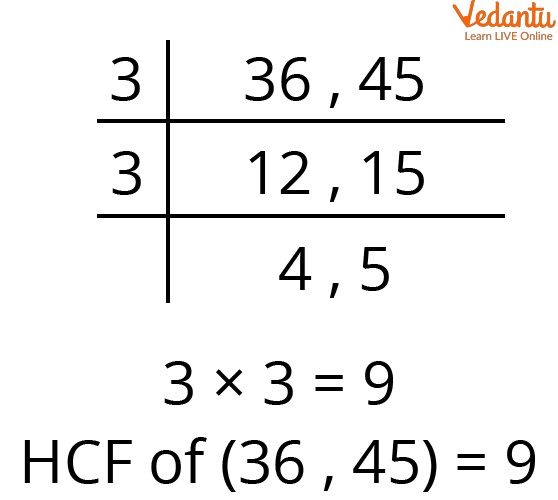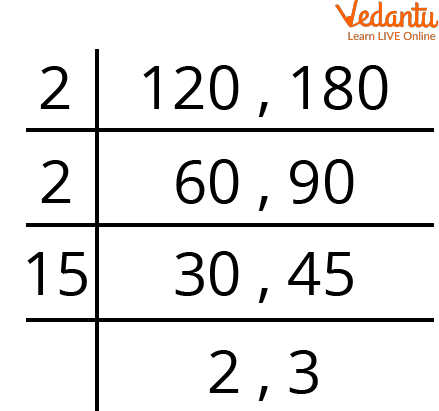Courses
Courses for Kids
Free study material
Offline Centres
More

# HCF QuestionsLast updated date: 27th Nov 2023
Total views: 144.6k
Views today: 3.44k## Introduction to HCF Problems

Highest Common Factor (HCF) as the name itself says, it's the method of finding the highest common factors of two or more than two numbers.  It's the highest common number which can divide the given two or more than two positive numbers equally.

To find the HCF, we first need to find out the factors of each given number.  Then we check for the common factors where the factors that we found out are the same and lastly we mark the highest factor of them all.

For example:

Let's take two positive numbers

1.  Find out the HCF of 6,12.

Ans:

• Step 1- Finding out the factorsFactors of 6,12

• Step 2: finding out the common factors

The factors of 6 and 12 are 1, 2, 3, 6

The Highest Common Factor of (6,12) = 6

## Understanding How to Find HCF Using Short Division Method

1.  Find out the HCF of 36 and 45.

Ans: The easiest method of finding out the HCF of large numbers is using the  short division method.

For this method, we need to find out the LCM (least Common Multiple) of 36 and 45.  Firstly draw a table and arrange the given numbers horizontally.  We need to find out the common factors which can divide the given number equally and put it on the left hand side.LCM Method to Find Out Common Factors

We keep on dividing until we get co-prime numbers at the end.  Prime number is a number that cannot be exactly divided by any other whole number other than itself and 1.

In this case. we got 4 and 5 which don't have common factors. Lastly, we multiply all the common factors that we have.

3X3 = 9

Therefore, the HCF of (36, 45) = 9.

## HCF Word Problem

1.  A milk seller has 120 liters of almond milk and 180 liters of soya milk.  He wants to sell the milk by filling both the kinds of milk in tins of equal amount.  Find the greatest volume of which the tin can take.

Ans: To find out the equal quantity of both the milks that the tin can take, we need to find out the factors of 120 and 180;

Well the target of the question is to find out the greatest volume of milks filled in the tins.  So, we have to find the largest number which exactly divides 120 and 180 equally which means  we will have to find out the HCF of 120 and 180.

Using LCM to find out the common factorsLCM of 120, 180

HCF of (120, 180)=> 2X2X15 = 60

Therefore, the greatest volume that the tins can take is 60 liters of each.

Which means 120 ÷ 60 = 2 tins of almond milk

180 ÷ 60 = 3 tins of soya milk.

## Practice Questions

1. Sneha has 40 strawberries and Eisha has 95 blueberries.  They want to distribute the fruits in such a way that each box has an equal number of fruits and also each box should have the exact set of strawberries or blueberries.  What is the greatest number of fruits that can be arranged in each box?

Ans: HCF of (40,95)= 5

Therefore, the fruits in the boxes can be arranged equally in the set of 5.

2. Two wooden boards are 77 m and 44 m long respectively.  The wooden boards are to be cut into pieces of equal length.  Find the maximum length of each board.

Ans: HCF (77,43)= 11

Therefore, the wooden boards can be cut into 11 equal lengths.

## Summary

In this article we learnt that HCF (highest common factor) also known as GCD (greatest common divisor) basically is the number which can divide the given two or more numbers equally and one of the easiest ways of finding out the HCF of large numbers is using the Short division method.  The article tries to explain the short division method step-by-step properly.

## FAQs on HCF Questions

1. Why is HCF calculated and what is its importance?

Although HCF is mainly used in mathematical problems to split things into smaller sections. It could be used to arrange something to rows and groups for distribution. It is/can be applied in our daily lives as well.  It can be used in real-time scenarios such as buying return gifts for the kids, donating food to the orphans, interior decor requirements etc.  There are several methods through which we can calculate HCF which are (1) prime factorization method (2) factorization method (3) short division method.

2. Can HCF of any given number be 0 or 1?

It is obviously possible that the HCF of the given figures can be 1 only if the given figures don’t have any other common factors apart from 1.  For example: HCF of (2,3)= 1,  HCF of (3,4)= 1, HCF of (4,5)= 1, HCF of (5,6)= 1, HCF of (9,10)= 1.  It is possible to have HCF of given figures to have 0 only if all the given figures are 0 because every natural number is a common divisor of 0 and 0, and therefore HCF (0,0)= 0.